## Triangle Center

A triangle center is a point whose Trilinear Coordinates are defined in terms of the side lengths and angles of a Triangle. The function giving the coordinates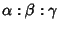is called the Triangle Center Function. The four ancient centers are the Centroid, Incenter, Circumcenter, and Orthocenter. For a listing of these and other triangle centers, see Kimberling (1994).

A triangle center is said to be Regular Iff there is a Triangle Center Function which is a Polynomial in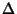,,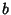, and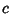(whereis the Area of the Triangle) such that the Trilinear Coordinates of the center are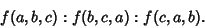A triangle center is said to be a Major Triangle Center if the Triangle Center Function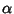is a function of Anglealone, and therefore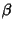and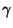of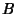and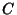alone, respectively.

See also Major Triangle Center, Regular Triangle Center, Triangle, Triangle Center Function, Trilinear Coordinates, Trilinear Polar

References

Davis, P. J. The Rise, Fall, and Possible Transfiguration of Triangle Geometry: A Mini-History.'' Amer. Math. Monthly 102, 204-214, 1995.

Dixon, R. The Eight Centres of a Triangle.'' §1.5 in Mathographics. New York: Dover, pp. 55-61, 1991.

Gale, D. From Euclid to Descartes to Mathematica to Oblivion?'' Math. Intell. 14, 68-69, 1992.

Kimberling, C. Central Points and Central Lines in the Plane of a Triangle.'' Math. Mag. 67, 163-167, 1994.

Kimberling, C. Triangle Centers and Central Triangles.'' Congr. Numer. 129, 1-295, 1998.